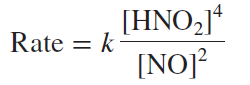# Problem: By what factor does the rate shown below change if each of the following changes occurs: (c) [HNO2] is halved?

###### FREE Expert Solution
80% (366 ratings)
###### Problem Details

By what factor does the rate shown below change if each of the following changes occurs:

(c) [HNO2] is halved?Frequently Asked Questions

What scientific concept do you need to know in order to solve this problem?

Our tutors have indicated that to solve this problem you will need to apply the Rate Law concept. You can view video lessons to learn Rate Law. Or if you need more Rate Law practice, you can also practice Rate Law practice problems.

What textbook is this problem found in?

Our data indicates that this problem or a close variation was asked in Chemistry: The Molecular Nature of Matter and Change - Silberberg 8th Edition. You can also practice Chemistry: The Molecular Nature of Matter and Change - Silberberg 8th Edition practice problems.